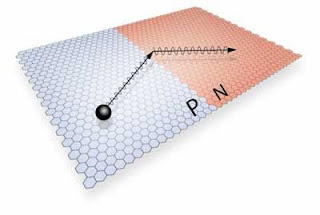# Learning about P-N Junction in briefHey, using phones or laptops, maybe there’s a little bit of concept of p-n junction involved there!!!

Let’s take a pore over in the world of the p-n junction.

Starting with the definition-

A semiconductor material (such as silicon, or germanium) is taken and doped with donor impurities (like Antimony, Arsenic, and Phosphorus) on one side and with acceptor impurities (like Indium, Boron, and Aluminum) on the other, this whole of the constructed structure is nothing but P-n junction.

Here is the diagram that shows the physical structure of a typical p-n junction which will help to understand the concept in a detailed manner:

The p-n junction diodes are constructed by doping the semiconductor plate with donor impurities making it n-type (excess of electrons). This doping is done from one side. At this point in the process there gets created a difference in electrons. That is the side from where the semiconductor was doped has a higher concentration of negatively charged carriers i.e., the electrons and on the other side, there is a low concentration of electrons. Therefore, there is a diffusion of electrons from the side of higher concentration to lower concentration. This further creates a side of an excess of negative charge carriers (electrons are in excess – n-type) and a side with an excess of positive charge carriers (holes are in excess – p-type). Thus, this is the process behind the construction of the p-n junction.

Now this state, where the positive charge carriers (holes) and negative charge carriers (electrons) co-exist adjacently, is not the stable state.

Come next…. what happens now

Formation of Depletion region-

There is a tendency of charge carriers to move from higher to a lower concentration that is if it is the n-side the electrons have a higher density at this side when compared to the p-side, so some electrons start diffusing towards the p-side (right side as per the above diagram). This same process takes place with holes on the p-side, they diffuse towards the left side. As the two halves (left-half and right-half) were electrically neutral in the beginning, diffusion of holes towards the left and diffusion of electrons towards the right makes the left half positively charged and the right half negatively charged. Thus, an electric field gets developed near the junction directed from the left to the right side. Any hole near the junction gets pushed towards the right half and an electron near it gets pushed towards the right side. Therefore, no charge carrier can remain in a small region near the junction the region is called the depletion region.

Diffusion current-

Charge carriers trying to move from higher to lower concentration for example if it is n-side the electrons have a higher density at this side so it will try to move towards the p side. But due to the presence of depletion region only holes and electrons with high kinetic energy can pass through this region.

(diffusion current density due to holes) Jp=-eDp(dp/dx)

(diffusion current density due to electrons) Jn=+eDn(dn/dx)

Thus, here comes the role of the Potential Barrier’s presence in the depletion layer allows a small amount of diffusion.

But some holes and electrons have enough energy to cross the potential barrier. Thus, diffusion results in an electric current called diffusion current from to p-side to n-side.

Drift current- The flow of drift current occurs due to the electric field which was generated in the depletion region. The electrons regularly flow to the n-side and holes towards the p-side. This generates an electric current from the n-side to the p-side. Now, this drift current is in the opposite direction to the diffusion current which was from p-side to n-side.

The neutrality of p-n junction

In steady-state, the diffusion current equals drift current in magnitude and there is no net transfer of charge at any cross-section.

So, that’s all.